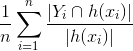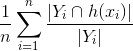2 views

I'm wondering how to calculate precision and recall measures for multiclass multilabel classification, i.e. classification where there are more than two labels, and where each instance can have multiple labels?

by (33.1k points)

Multiclass classification:  It is a classification task with more than two classes; e.g., classify a set of images of fruits which may be oranges, apples, or pears. Multiclass classification makes the assumption that each sample is assigned to one and only one label: a fruit can be either an apple or a pear but not both at the same time.

Multilabel classification: It assigns to each sample in a set of target labels. This can be thought of as predicting properties of a data-point that are not mutually exclusive, such as topics that are relevant for a document.

The metrics are computed in a per datapoint manner. For each predicted label, only the score is computed, and then these scores are aggregated over all the data points.

Precision:

It calculates the proportion of positive predictions, those are actually correct.Recall:
It calculates the proportion of actual positives that were identified correctly.To calculate precision and recall for multiclass-multilabel classification. You can add the precision and recall separately for each class, then divide the sum with the number of classes. You will get the approximate calculation of precision and recall for them.

If you wish to learn more about Machine Learning, visit Machine Learning tutorial and Machine Learning online course by Intellipaat.